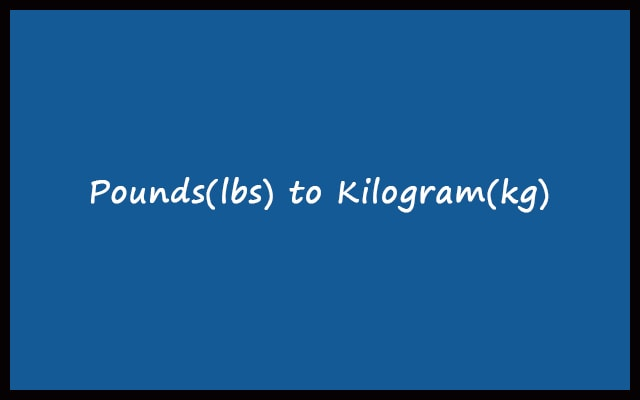# lbs to kg – Pounds to Kilograms Converter Calculator

Pounds and kilograms are the most used weight measuring units. To convert pounds to kilograms use our lbs to kg converter online. Also, it just takes less than a second for conversion.

Formula: 1 lb = 0.45359237 kg
 Enter Pounds: Pounds + Ounces: lb oz Kilograms: Kilograms + Grams: kg g Calculation
RelatedBoth Kilogram (kg) and Pound (lbs) are using to record weight. Have you ever weighed yourself? If your weight measuring device gives reading in pounds. So, you can change it into kilograms (kg) easily with our lbs to kg converter. This is the best effective way to convert from pounds to kg in seconds.

In this article, we’ll know about the tool. Along with mathematics calculation behind pounds to kg. Lastly, the advantages and procedures to use the tool. These are the contents. Firstly, let’s get some general knowledge of Pounds and kilograms.

### Pounds (lb)

Pounds is symbolized by ‘lb’. Your geographical location is an important factor. In order to decide what weight unit you use. If you are from the United States and the United Kingdom. Then the pound holds the majority. To measure weight or mass, they prefer pounds. The kilogram is used rarely. If you are not from the U.S or U.K. Then you may mainly prefer Kilogram. However, if you want to kg from pounds. Use the tool to convert pounds to kg. The Roman Libra is the reason for the conceptualization.

There is also a smaller unit than the pound associated with it known as “ounces”. The ounce is symbolized by “oz” just like Kilogram and Gram. Even more, you can check How many ounces in a pound here.

### Kilogram (kg)

The kilogram is the SI unit to measure mass. Also, it is symbolizing by ‘kg’. For pure water, the mass of 1000 cm3 water is 1 kg. It was a conventional definition of the kilogram. The modern definition of a kilogram is based on the prototype. The International Prototype consists of Platinum and Iridium. You can get the actual prototype at the International Bureau of Weights and Measures.

## How to convert lbs to kg?

To interchange one unit to another. The most important thing is to know the relationship between both units. Hence, the relationship between lbs and kg is:

##### 1 Pound = 0.45359237 kilograms

So, if you want to convert pounds to kg. Then just multiply the pound value with 0.45359237. The equation will look like:

##### M(kg) = lbs x 0.45359237 kg

So, Let’s look at some examples to understand.

#### Example 1: Convert 20 lbs to kg.

Solution:

Here, pounds = 20 lbs

We know that,

Kg = lbs x 0.45359237 Kg

or, Kg = 20 x 0.45359237 = 9.0718474 kg

Hence, 20 pounds = 9.0718474 Kg

#### Example 2: Convert 100 pounds to kilograms.

Solution:

Here, pounds = 100 lbs

So, Kg = 100 x 0.45359237 = 45.359237 kg

Hence, 100 pounds = 45.359237 Kg

#### Example 3: Convert 0.862 pounds to kg.

In this case, Pounds = 0.862 lbs

Kg = 0.862 x 0.45359237 = 0.39099662294 kg

Hence, 0.862 pounds = 0.39099662294 Kg

### Advantages of using lbs to kg Converter

1. The pounds into kilogram converter is simple to use. Anyone can use it with very ease.
2. You can enter directly value in the pound. Otherwise, enter in pounds and ounces. The output will be display in Kg and grams.
3. Even more, this converter or calculator is very fast. While going for a manual calculation. It can take a lot of time. Go for the converter to save time.
4. It is a handy tool thus can be used anywhere anytime. In Short, using web applications is efficient. It helps us all the time when the pen-paper may not available. Also, you may not always remember the conversion equation.
5. The web application converter is 100% accurate. So, don’t worry about getting an incorrect answer. It follows the pre-defined path for all inputs.

### How to use the Pounds to Kg Converter Calculator?

• The lb into kg converter is an online tool. Hence, you need an Internet connection. Along with a device to access. After that follow the next steps.
• Enter your pound figure in the input. For also entering ounces. Go to the pounds + ounces section. Fill the input box accordingly.
• To convert pounds to kilogram click on “Convert”.

As a result, the output will be displayed below in kilograms as well as kg + gram. For new conversion just click on “Reset”. Also, check out our Kg to lbs converter.

#### Pounds to Kilograms conversion table

Pounds (lb) Kilograms (kg)
0 lb 0 kg
0.1 lb 0.045 kg
1 lb 0.454 kg
2 lb 0.907 kg
3 lb 1.361 kg
4 lb 1.814 kg
5 lb 2.268 kg
6 lb 2.722 kg
7 lb 3.175 kg
8 lb 3.629 kg
9 lb 4.082 kg
10 lb 4.536 kg
20 lb 9.072 kg
30 lb 13.608 kg
40 lb 18.144 kg
50 lb 22.680 kg
60 lb 27.216 kg
70 lb 31.751 kg
80 lb 36.287 kg
90 lb 40.823 kg
100 lb 45.359 kg
1000 lb 453.592 kg